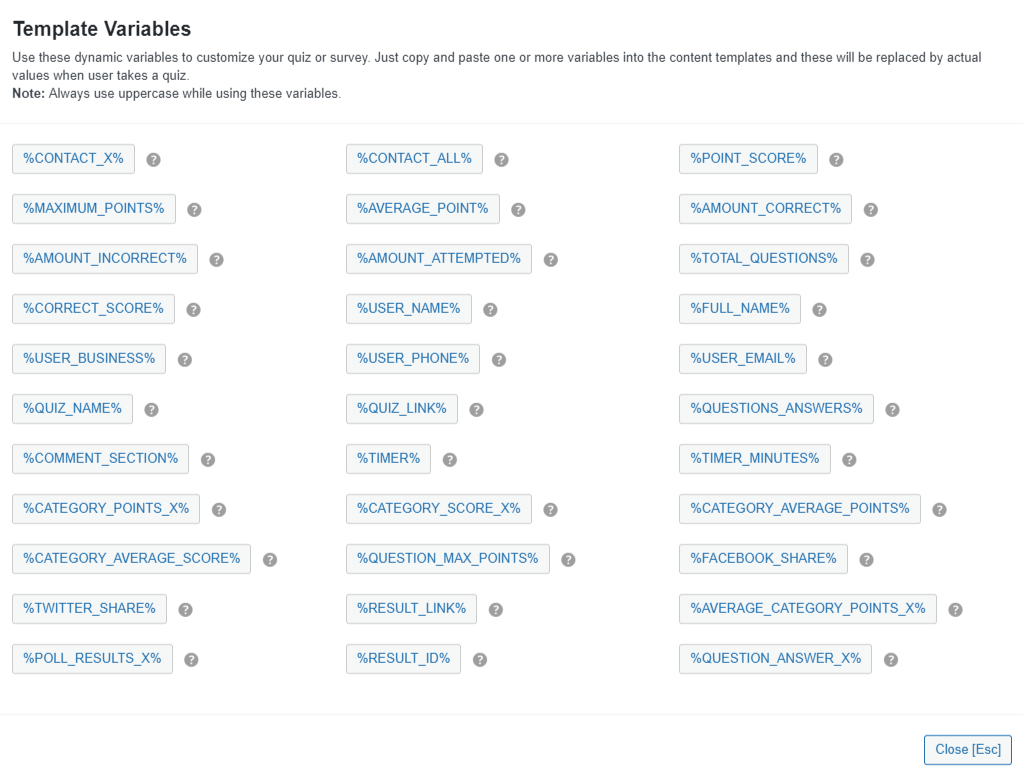# Template VariablesQuiz and Survey Master uses a template variable system for all the text that you will customize. This system allows you to put a variable in your template and that variable be replaced with the corresponding value when the user takes the quiz. For example, when we write the results page template, we won’t be able to put the points the user has earned because the user hasn’t taken the quiz yet. So, we can put the variable %POINT_SCORE% on the results page. Then, when the user takes the quiz, the plugin will put the total points in place of the variable for you.

For example, in our Twitter Sharing Text template, we might have put “I just scored %CORRECT_SCORE%% on %QUIZ_NAME%!”. So, when the quiz is taken and the user tweets this, it will become something like this: “I just scored 87% on Frank’s Test Quiz!”.

There are lots of variables you can use in your templates. Each template includes a list of variables that work in that template. For example, the Message Displayed Before Quiz template can only have the variables %QUIZ_NAME% and %CURRENT_DATE%. So be sure to check which variables are allowed before you write that masterpiece in the template.

For a complete list of variables and how to use them, keep reading. Or, now that we understand the basics, let’s create our first quiz!

%CONTACT_X% – This is used to show the value user entered into the contact field. Where X is the number of contact field. For example, the first contact field would be %CONTACT_1%. Check the below example:

`Great Job %CONTACT_1% Stay connected for more!`

%CONTACT_ALL% – this is used to list the user values for all the contact fields entered by the user. For example:

`Great Job!These are the details you entered %CONTACT_ALL%`

%POINT_SCORE% – this is used to show the number of points a user earned during the quiz. For example:

`Great job! You earned %POINT_SCORE% points!`

%MAXIMUM_POINTS% – This is used to display the maximum possible points one can score in the given test/quiz. For Example:

`Welcome! This test is of %MAXIMUM_POINTS% points. Good Luck!`

%AVERAGE_POINT% – this is the average amount of points the user earned per question. For example:

`Great Job! You averaged %AVERAGE_POINT% points per question!`

%AMOUNT_CORRECT% – the number of questions the user answered correctly. For example:

`You answered %AMOUNT_CORRECT% questions correctly!`

%AMOUNT_INCORRECT% – the number of questions the user answered incorrectly. For example:

`You answered %AMOUNT_INCORRECT% questions incorrectly!`

%AMOUNT_ATTEMPTED% – The total number of questions attempted by the user. For Example:

`You attempted %AMOUNT_ATTEMPTED% questions in this test.`

%TOTAL_QUESTIONS% – the total amount of questions that were displayed for the user. For example:

`The quiz you just took had %TOTAL_QUESTIONS% questions!`

%CORRECT_SCORE% – the percentage score the user earned during the quiz. For example:

`Great job! You scored %CORRECT_SCORE% % on this quiz!`

%USER_NAME% – the name the user entered into the Name text box. For example:

`Thank you %USER_NAME% for taking this quiz!`

%FULL_NAME% – This will display the full name entered by the user before the quiz began. For Example:

`%FULL_NAME% you have successfully taken the online test.`

%USER_BUSINESS% – the company the user entered into the Business text box. For example:

`%USER_NAME% from the company of %USER_BUSINESS% just took this quiz!`

%USER_PHONE% – the phone number the user entered into the Phone text box. For example:

`%USER_NAME% just took the quiz. This user can be reached at %USER_PHONE%.`

%USER_EMAIL% – the email the user entered into the Email text box. For example:

`%USER_NAME% just took the quiz and can be emailed at %USER_EMAIL%`

%QUIZ_NAME% – the name of the quiz. For example:

`Great Job %USER_NAME%! You have just taken the %QUIZ_NAME%.`

%QUIZ_LINK% – this will display the link of the current quiz. For Example:

`You are free to share this link with your friends %QUIZ_LINK%`

%QUESTIONS_ANSWERS% – shows the questions, answers, and comments. This variable can be edited on the Text tab. An example of usage would be:

```Great Job %USER_NAME%! You earned %POINT_SCORE% points. Review your answers below to see which questions you answered correctly and which ones you did not.

%COMMENT_SECTION%  – the text the user entered into the quiz comment section (if enabled). An example of usage would be:

```%USER_NAME% took the quiz and left these comments:
%COMMENT_SECTION%```

%TIMER_MINUTES% – This is used to show the amount of time taken by the user on the quiz in minutes.

`Hi %USER_NAME%, you took %TIMER_MINUTES% minutes to complete the quiz.`

%TIMER% – the number of seconds the user took to complete the quiz. For example:

`The user took %TIMER% seconds to complete this test.`

%CATEGORY_POINTS_X%–  this is used to display the number of points a user earned in a specific category. An example of the usage would be:

`You earned %CATEGORY_POINTS_History% points in the History category.`

%CATEGORY_SCORE_X% – this is used to display the percentage score of questions the user answered correctly in a specific category. For example:

`You earned %CATEGORY_SCORE_Math% in the Math category of this quiz.`

%CATEGORY_AVERAGE_POINTS% – this is used to display the average amount of points the user earned between all categories.For Example:

`On average, you earned %CATEGORY_AVERAGE_POINTS% points in every category for this quiz.`

%CATEGORY_AVERAGE_SCORE% – this is used to display the average percentage score of questions the user answered in all categories. For example:

`On average, you earned %CATEGORY_AVERAGE_SCORE% % per category for this quiz.`

%QUESTION_MAX_POINTS% – This will display the Maximum points allotted to the question. This variable will work in the loop, you can use this variable in the `Text` tab. For Example:

`You have earned %QUESTION_MAX_POINTS% points for the asked question.`

```Be sure to share your results on Facebook by using the button below!

```Be sure to share your results on Twitter by using the button below.

%RESULT_LINK% – This will display the link of the results page. For Example:

`Thanks for taking this exam, here is the results link: %RESULT_LINK%`

%AVERAGE_CATEGORY_POINTS_X% – Here “X” is the Category name. This will display the average amount of points a specific category earned. For Example:

`Awesome! Your Average Category Score was %AVERAGE_CATEGORY_POINTS_X%.`

%POLL_RESULTS_X% – this is used to display the poll results bearing a particular question ID. Note: It’s only supported for multiple choice answers. For Example:

`Here are the Poll results %POLL_RESULTS_1%`

%RESULT_ID% – this is used to show the result id. That is the result number. For Example:

`The result number for this quiz is: %RESULT_ID%`

%QUESTION_ANSWER_X% – this is used to display the result of a particular question. Where ‘X‘ is the Question ID. Question ID can be found in the Edit Question Window in the Questions Tab. For Example:

`Your response for Question 10 was %QUESTION_ANSWER_10%`